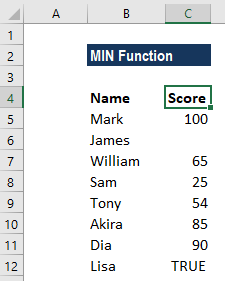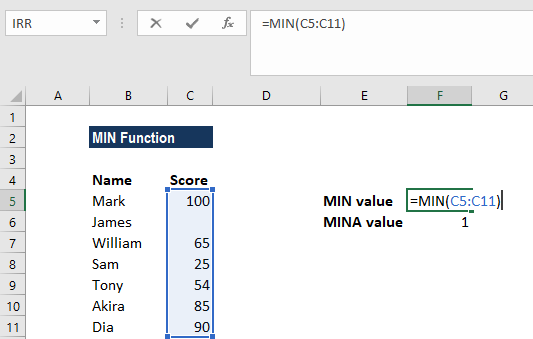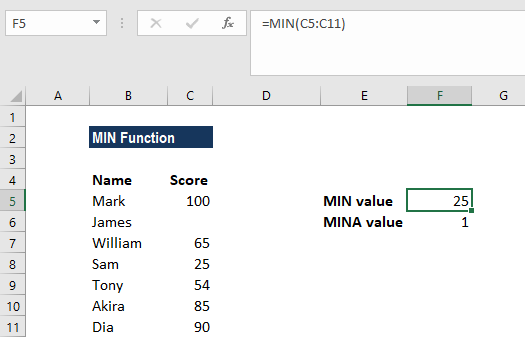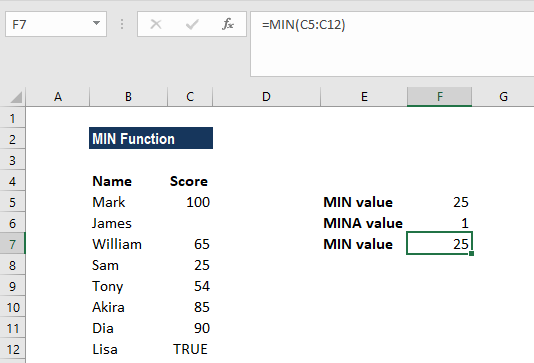# MIN Function

Returns the minimum value in a given list of arguments

## What is the MIN Function?

The MIN function is categorized under Excel Statistical functions. MIN will return the minimum value in a given list of arguments. From a given set of numeric values, it will return the smallest value. Unlike the MINA function, the MIN function ignores numbers, text, and logical values TRUE and FALSE and text values.

In financial modeling, the MIN formula can be useful in calculating debt schedules and depreciation schedules.

### Formula

=MIN(number1, [number2], …)

Number1 and number2 are the arguments used for the MIN function, where Number1 is required and the subsequent values are optional.

In MS Excel 2007 and later versions, we can provide up to 255 number arguments to the MIN function. However, in Excel 2003 and earlier versions, it can only accept up to 30 number arguments.

Arguments can be provided as constants, or as cell references or ranges. If an argument is supplied to the function as a reference to a cell, or an array of cells, the MIN function will ignore blank cells and text or logical values contained within the supplied cell range. However, logical values and text representations of numbers that are supplied directly to the function will be included in the calculation.

### How to use the MIN Function in Excel?

As a worksheet function, the MIN function can be entered as part of a formula in a cell of a worksheet. To understand the uses of the function, let us consider an example:

#### Example

Let us calculate the lowest marks from the following data:The formula used was:The MIN function ignored the empty value and returned 25 as the result.As seen above, MIN will ignore empty values. In this example, if we provide a logical value, the function will ignore it and will give the same result, but MINA will consider it, as shown below:### A few things to remember about the MIN Function

1. #VALUE! error – Occurs if any values that are provided directly to the MIN function are non-numeric.
2. The primary difference between MIN and MINA is that MINA evaluates TRUE and FALSE values as 1 and 0, respectively. Hence, if we wish to include logical values, we need to use the MINA function.

Thanks for reading CFI’s guide to important Excel functions! By taking the time to learn and master these functions, you’ll significantly speed up your financial analysis. To learn more, check out these additional CFI resources:

• Excel Functions for Finance